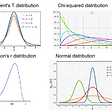# Generate Random Data

`x <- rnorm(1000,1000,20) m <- 6 c <- 10 y <- m*x + c   simdf <- data.frame(x = x , y=y)`
• This is what the data looks like
`head(simdf)## x y ## 1 1005.4237 6042.542 ## 2 1009.2766 6065.660 ## 3 1001.2252 6017.351 ## 4 995.0024 5980.014 ## 5 964.0087 5794.052 ## 6 979.7846 5888.707`
• Sampling few rows from our “Population”
`sampSize <- 100 sample1 <- simdf[sample(seq(1 , nrow(simdf) , 1) , sampSize ),] sample2 <- simdf[sample(seq(1 , nrow(simdf) , 1) , sampSize ),]  head(sample1)## x y ## 440 970.4631 5832.779 ## 48 1011.4937 6078.962 ## 199 1006.4098 6048.459 ## 723 992.0803 5962.482 ## 375 999.7168 6008.301 ## 705 978.0303 5878.182head(sample2)## x y ## 336 1024.1023 6154.614 ## 476 1005.8980 6045.388 ## 439 1024.4581 6156.749 ## 157 972.8277 5846.966 ## 30 993.1578 5968.947 ## 233 994.1998 5975.199`

# Are these two samples from the same distribution?

• what if we didn’t know already

`s1mean <- mean(sample1\$y) s2mean <- mean(sample2\$y)  print(s1mean)##  5997.703print(s2mean)##  6009.034`

# Is the difference between the means actually statistically significant

• Differences of means will be normally distributed if we repeatedly sample.

• Sampling distribution of difference of means will be normally distributed

• But since we don’t know the distribution parameters

• We assume T-distribution

# Two sample T-test

`##  “combined sd of the t distribution”##  15.78279## t_stat t_crit degf ## 1 -0.7179117 1.652648 196.4361t.test(sample1\$y , sample2\$y)##  ## Welch Two Sample t-test ##  ## data: sample1\$y and sample2\$y ## t = -0.71791, df = 196.44, p-value = 0.4737 ## alternative hypothesis: true difference in means is not equal to 0 ## 95 percent confidence interval: ## -42.45612 19.79481 ## sample estimates: ## mean of x mean of y  ## 5997.703 6009.034data.frame(fstat = sd(sample1\$y)²/sd(sample2\$y)²  , fcrit = qf(0.95 , df1 = nrow(sample1) , df2 = nrow(sample2)))## fstat fcrit ## 1 1.195933 1.39172var.test(sample1\$y , sample2\$y)##  ## F test to compare two variances ##  ## data: sample1\$y and sample2\$y ## F = 1.1959, num df = 99, denom df = 99, p-value = 0.3749 ## alternative hypothesis: true ratio of variances is not equal to 1 ## 95 percent confidence interval: ## 0.8046737 1.7774364 ## sample estimates: ## ratio of variances  ## 1.195933`

# Conclusions

• We can see that these two samples have similar means and variances therefore its safe to assume they come from the same distribution/population

`summary(aov(sample1\$y ~ sample2\$y))`## Df Sum Sq Mean Sq F value Pr(>F)  ## sample2\$y 1 139428 139428 11.35 0.00108 ** ## Residuals 98 1203617 12282  ## — - ## Signif. codes: 0 ‘***’ 0.001 ‘**’ 0.01 ‘*’ 0.05 ‘.’ 0.1 ‘ ‘ 1`

--

--

--

## More from Abhijeet Pokhriyal

School of Data Science @ University of North Carolina — Charlotte

Love podcasts or audiobooks? Learn on the go with our new app.## Abhijeet Pokhriyal

School of Data Science @ University of North Carolina — Charlotte

## Rapid revision notes: K Nearest Neighbors Algorithm## Lazy Predict-Intro and error## Hypothesis testing for dummies